• 2017-11-13 16:32:26

1、遗传算法

2、粒子群算法

3、模拟退火

4、蚁群算法

更多相关内容
• 遗传算法以一种群体的所有个体为对象，并利用随机化...参数编码、初始群体的设定、适应度函数的设计、遗传操作设计、控制参数设定五个要素组成了遗传算法的核心内容，此程序利用Python实现遗传算法求函数最值问题。
• MATLAB，使用遗传算法（GA算法某个函数的最小值，最基础的遗传算法实现
• 一个遗传算法的实例，用matlab写的，包含画图，求解一元函数的最大值。
• 各种智能算法程序以函数最值为例-蚁群算法.rar 首先声明，程序部分为原创，部分网络下载，部分为根据他人成果做细微改动。 这是本人智能控制的小作业，主要是用多种智能算法进行函数优化，包括遗传算法，蚁群...matlab
• MATLAB遗传算法求函数最值 问题 y=(x(1)2+x(2)2)0.25*(sin((50*(x(1)2+x(2)^2 ))^0.1 )+1) 的最小值。 遗传算法 简单说，就是通过模拟种群遗传繁殖与自然选择找到最好的个体。 一般情况，我们只要能把数据转化成...

# MATLAB遗传算法求函数最值

## 问题

求 y=(x(1)2+x(2)2)0.25*(sin((50*(x(1)2+x(2)^2 ))^0.1 )+1) 的最小值。

## 遗传算法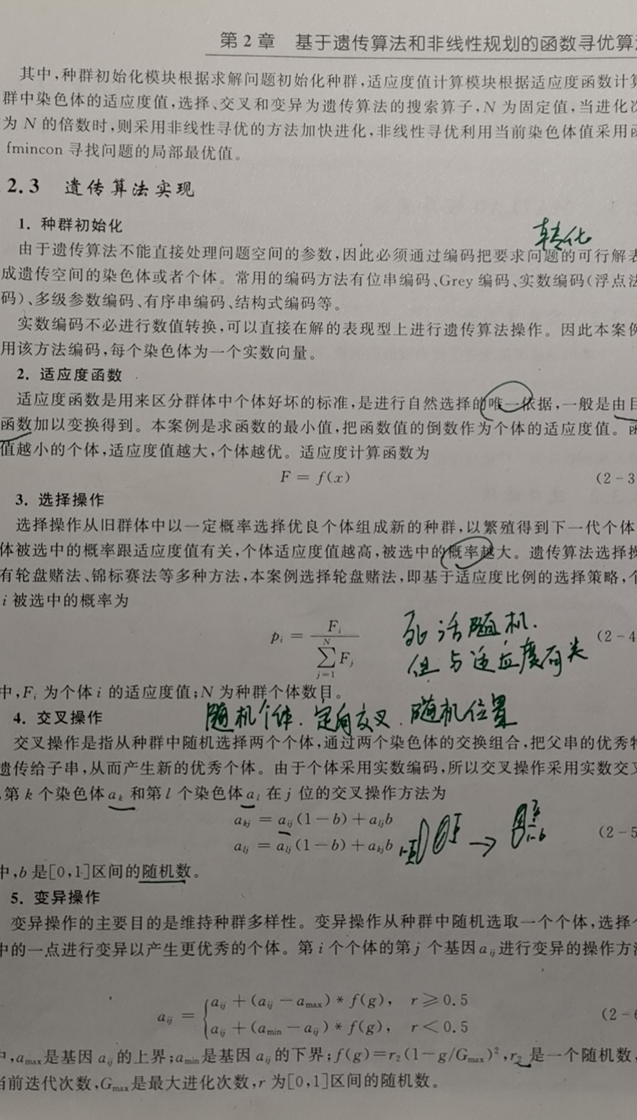简单说，就是通过模拟种群遗传繁殖与自然选择找到最好的个体。
一般情况，我们只要能把数据转化成矩阵、把自然语言转化成函数即可，并需要调整以下参数：

%% 遗传算法参数
maxgen=30;                         %进化代数
sizepop=100;                       %种群规模
pcross=[0.6];                      %交叉概率
pmutation=[0.1];                  %变异概率
lenchrom=[1 1 1 1 1];              %变量字串长度
bound=[0 0.9*pi;0 0.9*pi;0 0.9*pi;0 0.9*pi;0 0.9*pi];  %变量范围
x=fmincon(inline('-5*sin(x(1))*sin(x(2))*sin(x(3))*sin(x(4))*sin(x(5))-sin(5*x(1))*sin(5*x(2))*sin(5*x(3))*sin(5*x(4))*sin(5*x(5))'),chrom(i,:)',[],[],[],[],[0 0 0 0 0],[2.8274 2.8274 2.8274 2.8274 2.8274]);  %目标函数


合理情况：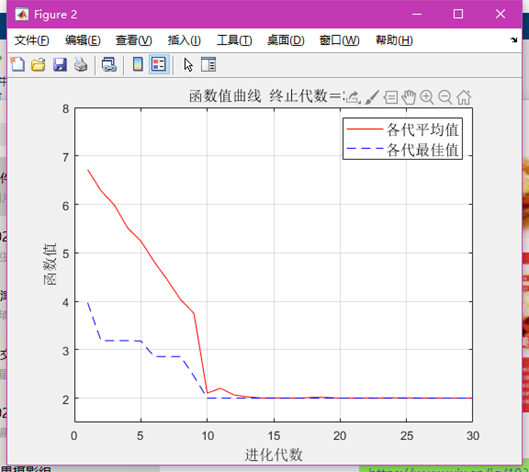代数太小：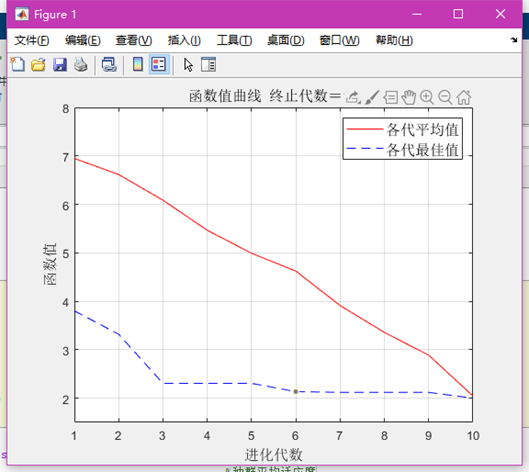种群太小：但代数或种群太大，程序运行会很慢。
交叉概率太小：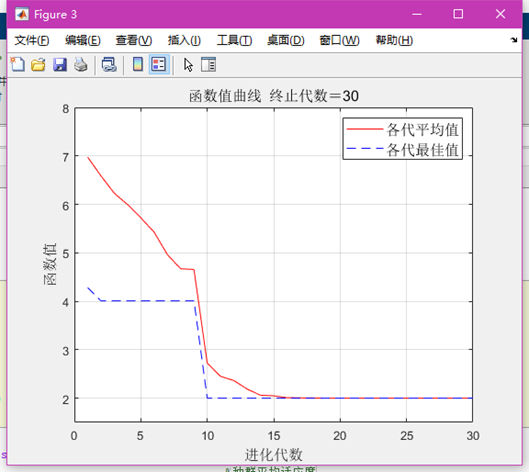变异概率太大（变异要存在，以防使进化方向错误）：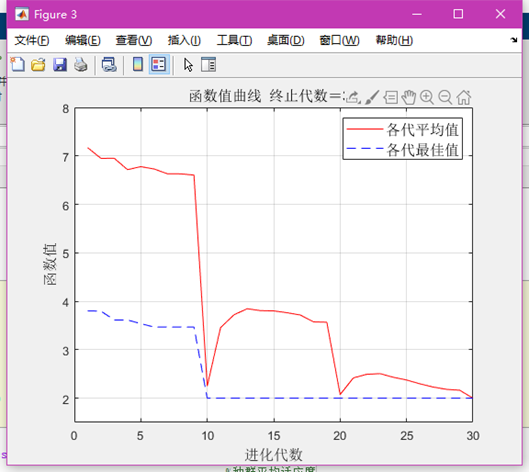## MATLAB代码

### Code.m

function ret=Code(lenchrom,bound)
%本函数将变量编码成染色体，用于随机初始化一个种群
% lenchrom   input : 染色体长度
% bound      input : 变量的取值范围
% ret        output: 染色体的编码值

flag=0;
while flag==0
pick=rand(1,length(lenchrom));
ret=bound(:,1)'+(bound(:,2)-bound(:,1))'.*pick; %线性插值
flag=test(lenchrom,bound,ret);             %检验染色体的可行性
end


### fun.m

function y = fun(x)

y=(x(1)^2+x(2)^2)^0.25*(sin((50*(x(1)^2+x(2)^2))^0.1)+1);


### test.m

function flag=test(lenchrom,bound,code)
% lenchrom   input : 染色体长度
% bound      input : 变量的取值范围
% code       output: 染色体的编码值

flag=1;
[n,m]=size(code);

for i=1:n
if code(i)<bound(i,1) || code(i)>bound(i,2)
flag=0;
end
end


### Cross.m

function ret=Cross(pcross,lenchrom,chrom,sizepop,bound)
%本函数完成交叉操作
% lenchrom              input  : 染色体的长度
% chrom                 input  : 染色体群
% sizepop               input  : 种群规模
% ret                   output : 交叉后的染色体

for i=1:sizepop

% 随机选择两个染色体进行交叉
pick=rand(1,2);
while prod(pick)==0
pick=rand(1,2);
end
index=ceil(pick.*sizepop);
% 交叉概率决定是否进行交叉
pick=rand;
while pick==0
pick=rand;
end
if pick>pcross
continue;
end
flag=0;
while flag==0
% 随机选择交叉位置
pick=rand;
while pick==0
pick=rand;
end
pos=ceil(pick.*sum(lenchrom)); %随机选择进行交叉的位置，即选择第几个变量进行交叉，注意：两个染色体交叉的位置相同
pick=rand; %交叉开始
v1=chrom(index(1),pos);
v2=chrom(index(2),pos);
chrom(index(1),pos)=pick*v2+(1-pick)*v1;
chrom(index(2),pos)=pick*v1+(1-pick)*v2; %交叉结束
flag1=test(lenchrom,bound,chrom(index(1),:));  %检验染色体1的可行性
flag2=test(lenchrom,bound,chrom(index(2),:));  %检验染色体2的可行性
if   flag1*flag2==0
flag=0;
else flag=1;
end    %如果两个染色体不是都可行，则重新交叉
end
end
ret=chrom;


### Mutation.m

function ret=Mutation(pmutation,lenchrom,chrom,sizepop,pop,bound)
% 本函数完成变异操作
% lenchrom              input  : 染色体长度
% chrom                 input  : 染色体群
% sizepop               input  : 种群规模
% pop                   input  : 当前种群的进化代数和最大的进化代数信息
% ret                   output : 变异后的染色体

for i=1:sizepop
% 随机选择一个染色体进行变异
pick=rand;
while pick==0
pick=rand;
end
index=ceil(pick*sizepop);
% 变异概率决定该轮循环是否进行变异
pick=rand;
if pick>pmutation
continue;
end
flag=0;
while flag==0
% 变异位置
pick=rand;
while pick==0
pick=rand;
end
pos=ceil(pick*sum(lenchrom));  %随机选择了染色体变异的位置，即选择了第pos个变量进行变异
v=chrom(i,pos);
v1=v-bound(pos,1);
v2=bound(pos,2)-v;
pick=rand; %变异开始
if pick>0.5
delta=v2*(1-pick^((1-pop(1)/pop(2))^2));
chrom(i,pos)=v+delta;
else
delta=v1*(1-pick^((1-pop(1)/pop(2))^2));
chrom(i,pos)=v-delta;
end   %变异结束
flag=test(lenchrom,bound,chrom(i,:));     %检验染色体的可行性
end
end
ret=chrom;


### select.m

function ret=Select(individuals,sizepop)
% 本函数对每一代种群中的染色体进行选择，以进行后面的交叉和变异
% individuals input  : 种群信息
% sizepop     input  : 种群规模
% opts        input  : 选择方法的选择
% ret         output : 经过选择后的种群

individuals.fitness= 1./(individuals.fitness);
sumfitness=sum(individuals.fitness);
sumf=individuals.fitness./sumfitness;
index=[];
for i=1:sizepop   %转sizepop次轮盘
pick=rand;
while pick==0
pick=rand;
end
for j=1:sizepop
pick=pick-sumf(j);
if pick<0
index=[index j];
break;  %寻找落入的区间，此次转轮盘选中了染色体i，注意：在转sizepop次轮盘的过程中，有可能会重复选择某些染色体
end
end
end
individuals.chrom=individuals.chrom(index,:);
individuals.fitness=individuals.fitness(index);
ret=individuals;


### Genetic.m

最后运行这个↓

%% 清空环境
clc
clear

%% 遗传算法参数
maxgen=30;                         %进化代数
sizepop=100;                       %种群规模
pcross=[0.6];                      %交叉概率
pmutation=[0.01];                  %变异概率
lenchrom=[1 1];                    %变量字串长度
bound=[-5 5;-5 5];                 %变量范围

%% 个体初始化
individuals=struct('fitness',zeros(1,sizepop), 'chrom',[]);  %种群结构体
avgfitness=[];                                               %种群平均适应度
bestfitness=[];                                              %种群最佳适应度
bestchrom=[];                                                %适应度最好染色体
% 初始化种群
for i=1:sizepop
individuals.chrom(i,:)=Code(lenchrom,bound);       %随机产生个体
x=individuals.chrom(i,:);
individuals.fitness(i)=fun(x);                     %个体适应度
end

%找最好的染色体
[bestfitness bestindex]=min(individuals.fitness);
bestchrom=individuals.chrom(bestindex,:);  %最好的染色体
avgfitness=sum(individuals.fitness)/sizepop; %染色体的平均适应度
% 记录每一代进化中最好的适应度和平均适应度
trace=[];

%% 进化开始
for i=1:maxgen

% 选择操作
individuals=Select(individuals,sizepop);
avgfitness=sum(individuals.fitness)/sizepop;
% 交叉操作
individuals.chrom=Cross(pcross,lenchrom,individuals.chrom,sizepop,bound);
% 变异操作
individuals.chrom=Mutation(pmutation,lenchrom,individuals.chrom,sizepop,[i maxgen],bound);

% 计算适应度
for j=1:sizepop
x=individuals.chrom(j,:);
individuals.fitness(j)=fun(x);
end

%找到最小和最大适应度的染色体及它们在种群中的位置
[newbestfitness,newbestindex]=min(individuals.fitness);
[worestfitness,worestindex]=max(individuals.fitness);
% 代替上一次进化中最好的染色体
if bestfitness>newbestfitness
bestfitness=newbestfitness;
bestchrom=individuals.chrom(newbestindex,:);
end
individuals.chrom(worestindex,:)=bestchrom;
individuals.fitness(worestindex)=bestfitness;

avgfitness=sum(individuals.fitness)/sizepop;

trace=[trace;avgfitness bestfitness]; %记录每一代进化中最好的适应度和平均适应度
end
%进化结束

%% 结果显示
[r c]=size(trace);
figure
plot([1:r]',trace(:,1),'r-',[1:r]',trace(:,2),'b--');
title(['函数值曲线  ' '终止代数＝' num2str(maxgen)],'fontsize',12);
xlabel('进化代数','fontsize',12);ylabel('函数值','fontsize',12);
legend('各代平均值','各代最佳值','fontsize',12);
ylim([-0.5 5])
disp('函数值                   变量');
% 窗口显示
disp([bestfitness x]);


## 参考

https://blog.csdn.net/u010451580/article/details/51178225
https://www.bilibili.com/video/BV1gs411Q7et
史峰. MATLAB智能算法30个案例分析[M]. 北京航空航天大学出版社, 2011.

展开全文matlab
• 基于matlab程序语言的蚁群算法求解函数最优值，供广大科研工作者和程序爱好者学习使用参考！
• Java我们可以通过编写算法的方式，也可以通过数组先排序再取值的方式来实现。下面以最大值为例，解释一下多种算法。 (1) 自行实现，快速査找最大值 先来看用快速査找法取最大值的算法，其代码如下： ...

对一批数据进行排序，然后找出其中的最大值或最小值，这是基本的数据结构知识。在Java中我们可以通过编写算法的方式，也可以通过数组先排序再取值的方式来实现。下面以求最大值为例，解释一下多种算法。

(1)  自行实现，快速査找最大值

先来看用快速査找法取最大值的算法，其代码如下：

<span style="font-size:18px;"> public static int max(Integer[] datas){
int max=datas;
for(int i:datas){
max=max>i?max:i;
}
return max;
}</span>

这是我们经常使用的最大值算法，也是速度最快的算法。它不要求排序,只要遍历一遍数组即可找出最大值。

(2)  先排序，后取值

对于求最大值，也可以采用先排序后取值的方式，同样比较简单，代码如下：

<span style="font-size:18px;">  public static int max2(int[] datas){
Arrays.sort(datas);
int max=datas[datas.length-1];
return max;
}</span>

从效率上来讲，当然是自己写快速査找法更快一些了，只用遍历一遍就可以计算出最大值。但在实际测试中我们发现，如果数组数量少于1万，两者基本上没有差别，在同一个毫 秒级别里，此时就可以不用自己写算法了，直接使用数组先排序后取值的方式。

如果数组元素超过1万，就需要依据实际情况来考虑：自己实现，可以提升性能；先排序后取值，简单，通俗易懂。排除性能上的差异，两者都可以选择，甚至后者更方便一些， 也更容易想到。

接着往下思考，如果要査找仅次于最大值的元素（也就是老二），该如何处理呢？要注 意，数组的元素是可以重复的，最大值可能是多个，所以单单一个排序然后取倒数第二个元素是解决不了问题的。

此时，就需要一个特殊的排序算法了，先要剔除重复数据，然后再排序。当然，'自己写算法也可以实现，但是集合类已经提供了非常好的方法，要是再使用数组自己写算法就显得 有点过时了。数组不能剔除重复数据，但Set集合却是可以的，而且Set的子类TreeSet还能 自动排序。代码如下：

<span style="font-size:18px;">public static int getSecond(Integer[] data){
List<Integer> list=Arrays.asList(data);
System.out.println(list.size());
TreeSet<Integer> set=new TreeSet<Integer>(list);
return set.lower(set.last());
}</span>

剔除重复元素并升序排列，这都由TreeSet类实现的，然后可再使用lower方法寻找小 干最大值的值。大家看，上面的程序非常简单吧？那如果是我们自己编写代码会怎么样？那至少要遍历数组两遍才能计算出老二的值，代码的复杂度将大大提升。

也许你会说，这个要求有点变态，怎么会有这样的需求？不，有这样的需求很正常，比如在学校按成绩排名时，如果一个年级有1200人，只要找出最高的三个分数（可不一定就 是3个人，也可能是多人），是不是就是这种情况呢？因此在实际应用中求最值，包括最大值、最小值、第二大值、倒数第二小值等，使用集合是最简单的方式，当然若从性能方面来 考虑，数组是最好的选择。

注意最值计算时使用集合最简单，使用数组性能最优。

展开全文• 各种智能算法程序以函数最值为例-遗传算法.rar 首先声明，程序部分为原创，部分网络下载，部分为根据他人成果做细微改动。 这是本人智能控制的小作业，主要是用多种智能算法进行函数优化，包括遗传算法，蚁群...matlab
• 各种智能算法程序以函数最值为例-鱼群算法.rar 首先声明，程序部分为原创，部分网络下载，部分为根据他人成果做细微改动。 这是本人智能控制的小作业，主要是用多种智能算法进行函数优化，包括遗传算法，蚁群...matlab
• #include<iostream> using namespace std; int main() { int a, b, c; cout << "please input two numbers:"; cin >> a >> b; c = min(a, b); cout <...using nam
#include<iostream>
using namespace std;
int main()
{
int a, b, c;
cout << "please input two numbers:";
cin >> a >> b;
c = min(a, b);
cout << "min=" << c << endl;
return 0;
}
#include<iostream>
using namespace std;
int main()
{
int a, b, c;
cout << "please input two numbers:";
cin >> a >> b;
c = max(a, b);
cout << "max="<< c << endl;
return 0;
}

展开全文• Python实现遗传算法求函数最值 详细源代码：GA.py 1、算法过程图解 2、详细过程举例说明 （1）待求解方程 （2）确定编码方案 主要是确定编码长度： def segment_length(self): length = [] precision =[] for...python 人工智能
• 一、遗传算法概述 遗传算法（Genetic Algorithm，GA）最早是由美国的 John holland于20世纪70年代提出,...求解较为复杂的组合优化问题时,相对一些常规的优化算法,通常能够较快地获得较好的优化结果。遗传算法已被人
• 不使用遗传算法工具箱，编程实现求解函数优化问题 F(x,y)=sin(3πx)cos(4 π y)+cos(5 π x*sin(2 π y)) max{F(x,y)}, x in [-1,3], y in [-1,3] min{F(x,y)}, x in [-1,3], y in [-1,3]
• 例：下述二元函数的最大值： (1) 个体编码 遗传算法的运算对象是表示个体的符号串，所以必须把变量 x1, x2 编码为一种符号串。本题，用无符号二进制整数来表示。因 x1, x2 为 0 ~ 7之间的整数， 所以分别用...
• 该实验使用模拟退火算法求取函数的最小值，matlab自编程实现。该实验可以观察到搜索点的过程，也可以自行修改参数。
• 本程序使用了遗传算法，用于计算一个一元或者二元函数的最大值问题，属于入门级别的一段程序，下载后可以直接运行，一起交流学习。
•数组 算法
• 用粒子群算法16个经典函数的最小最大值，界面友好，运行时会出现动态二维图来展现粒子群是如何运动来求最值
• Python遗传算法求一元函数最大值前言代码后记参考文献 前言 最近接触遗传算法，参考了众多例子，有些又不尽然对，所以自己边理解边修改，然后写出了下面这堆传说的屎山。。。 PS1：遗传算法原理啥的太多了，就不...
• 蚁群算法简单应用function [F]=F(x1,x2) %目标函数F=-(x1.^2+2*x2...Endfunction [maxx,maxy,maxvalue]=antcolony% 蚁群算法求函数最大值的程序ant=200; % 蚂蚁数量times=50; % 蚂蚁移动次数rou=0.8; % 信息素挥发系...
• 这个代码是三元函数极值上的代码上改写的，如果你看了我遗传算法求三元函数的代码，你就不用下了，代码大部分不变，改的不多，你基本自己就能改出来了。
• 前面的话：咋们还是用scipy.optimize吧 例函数: y = 0.5 x 2 − 20 x + 1 y=0.5x^{2}-20x +1 y=0.5x2−20x+1 该函数(0, 100)的最小值. 模拟退火(SA)程序，如下: import math import numpy as np def x_y(x):...模拟退火 python 优化
• 前提 ...伪算法 MINIMUM(A) min=A for i = 2 to A.length if min > A[i] min = A[i] return min MAXIMUM(A) max=A for i = 2 to A.length if max < A[i] max = A[i] returnpython
• 遗传算法求取目标函数F(s)=21.5+x1*sin(4*pi*x1)+x2*sin(20*pi*x2)最值，采用精英保留测量，结果准确。人工智能课程设计，自己原创，MATLAB调试通过。
• 下列二元函数的最大值，f(x1,x2)=x1^2+x2^2,x1与x2的取值区间为{0,1,2，...，7}...